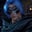Related Tags

communitycreator
python3
math

# How to check whether a number can be made a perfect squareGutha Vamsi Krishna

### Problem

Given two numbers n and k, the task is to check whether the given number n can be made a perfect square after adding k to it.

### Examples

• Input: n = 10, k = 6

• Output: YES

Explanation: $10 + 6 = 16$, which is a perfect square of $4$.

• Input: n = 11, k = 8

• Output: NO

Explanation: $11 + 8 = 19$, which is a not perfect square.

### Solution

• Add n and k (n+k), and then find the square root for n+k
• Check if num_sqrt*num_sqrt = n+k
• Print YES if it is a perfect square, otherwise print NO

### Implementation in Python

In the following code snippet, we:

• In line 1: Importing sqrt from module math to calculate square root.
• In line 3 to 4: Initialize n and k with 10 and 6 respectively.
• In line 6: Add k to n and store in num.
• In line 8: Calculating the square root of num.
• In line 10 to 13: Check for a perfect square.

It will print YES as $10 + 6 = 16$, square root $16$ is $4$, and satisfies the condition for perfect square as $4 * 4 = 16$.

from math import sqrt

#initialize N and K
N = 10
K = 6

num = N+K

#calculate square root
num_sqrt = int(sqrt(num))

#check for perfect square
if(num_sqrt * num_sqrt == num):
print("YES")
else:
print("NO")

The following code snippet also follows the same procedure as above except we changed the values for n and k.

It will print NO as $11 + 8 = 19$, which is not a perfect square. If we calculate square root for $19$, we get $4$, which doesn’t satisfy the condition for perfect square. $4 * 4 = 16$, not $19$.

from math import sqrt

#initialize N and K
N = 11
K = 8

num = N+K

#calculate square root
num_sqrt = int(sqrt(num))

#check for perfect square
if(num_sqrt * num_sqrt == num):
print("YES")
else:
print("NO")

RELATED TAGS

communitycreator
python3
math

CONTRIBUTORGutha Vamsi Krishna
RELATED COURSES

View all Courses

Keep Exploring

Learn in-demand tech skills in half the time